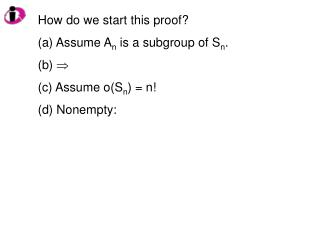DownloadDownload PresentationHow do we start this proof? Assume A n is a subgroup of S n .  Assume o(S n ) = n! Nonempty:

# How do we start this proof? Assume A n is a subgroup of S n .  Assume o(S n ) = n! Nonempty:

Télécharger la présentation## How do we start this proof? Assume A n is a subgroup of S n .  Assume o(S n ) = n! Nonempty:

- - - - - - - - - - - - - - - - - - - - - - - - - - - E N D - - - - - - - - - - - - - - - - - - - - - - - - - - -
##### Presentation Transcript

1. How do we start this proof? • Assume An is a subgroup of Sn. •  • Assume o(Sn) = n! • Nonempty:

2. What is the next step in the proof? • Closed: • Show An is a subgroup of Sn. • (c) Assume o(Sn) = n! • (d) Nonempty:

3. What is the next step in the proof? • Let a, b  H (b) Let f, g  An • (c) Let f, g  Sn (d) Let a*b  H • (e) Let f  g  An (f) Let f  g  Sn

4. What is the next step in the proof? • Identity: • Associative: • (c) Inverses: • (d) Nonempty:

5. What is the next step in the proof? • Let a  H (b) Let f  An • (c) Let f  Sn (d) Let a-1 H • (e) Let f -1  An (f) Let f -1  Sn

6. How many elements does S5 have? • 5 (b) 10 (c) 20 (d) 60 • (e) 120 (f) 200 (g) 500 (h) 546

7. How many elements does A5 have? • 5 (b) 10 (c) 20 (d) 60 • (e) 120 (f) 200 (g) 500 (h) 546